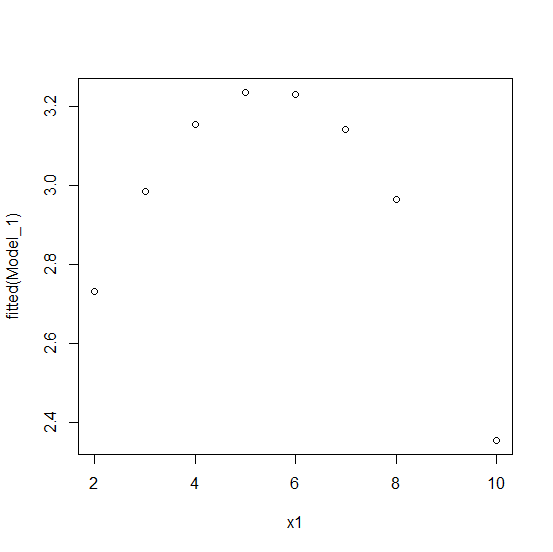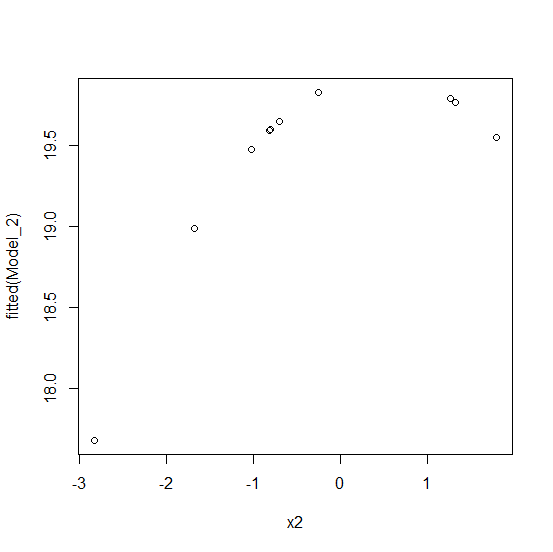How to create a plot of quadratic regression with fitted values against X in base R?

R ProgrammingServer Side ProgrammingProgramming

The quadratic regression model can be plotted by using the plot function but we would need to find the fitted values using the model and this can be done with the help of fitted function. For example, if we have a quadratic model M and the data has an independent variable x then the model against x can be created by using plot(x,fitted(M)).

Example

Live Demo

x1<-rpois(10,5)
y1<-rpois(10,2)
Model_1<-lm(y1~x1+I(x1^2))
plot(x1,fitted(Model_1))

OutputExample

Live Demo

x2<-rnorm(10)
y2<-rnorm(10,20,2.27)
Model_2<-lm(y2~x2+I(x2^2))
plot(x2,fitted(Model_2))

Output